# UNIT 7 Chemicals Reactions Types of Reactions Chemical

• Slides: 47UNIT 7: Chemicals Reactions & Types of Reactions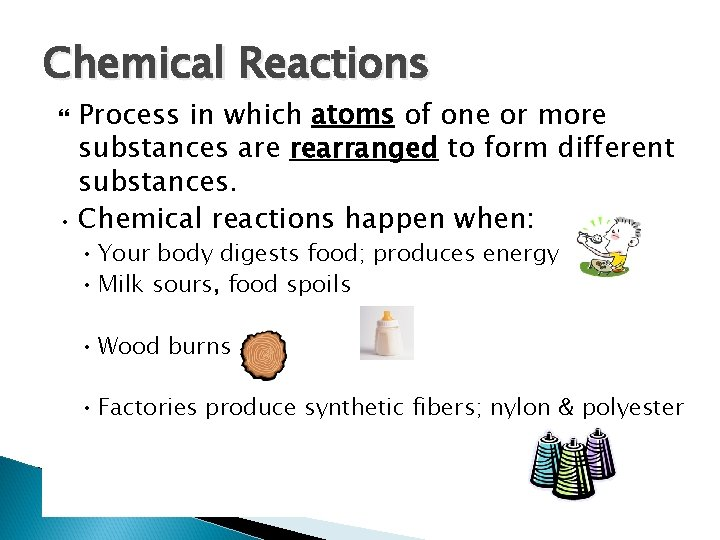Chemical Reactions Process in which atoms of one or more substances are rearranged to form different substances. • Chemical reactions happen when: • Your body digests food; produces energy • Milk sours, food spoils • Wood burns • Factories produce synthetic fibers; nylon & polyester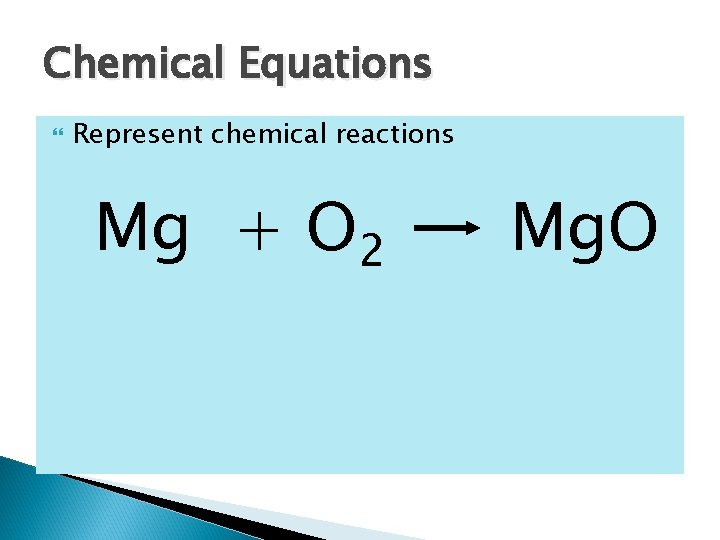Chemical Equations Represent chemical reactions Mg + O 2 Mg. O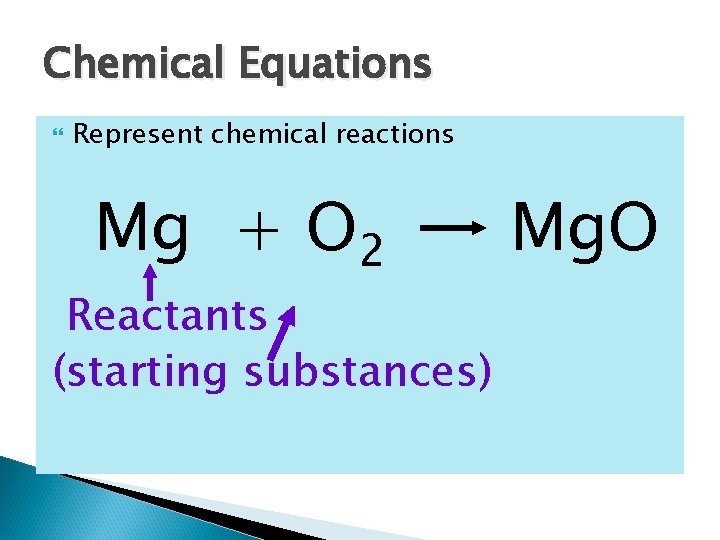Chemical Equations Represent chemical reactions Mg + O 2 Reactants (starting substances) Mg. O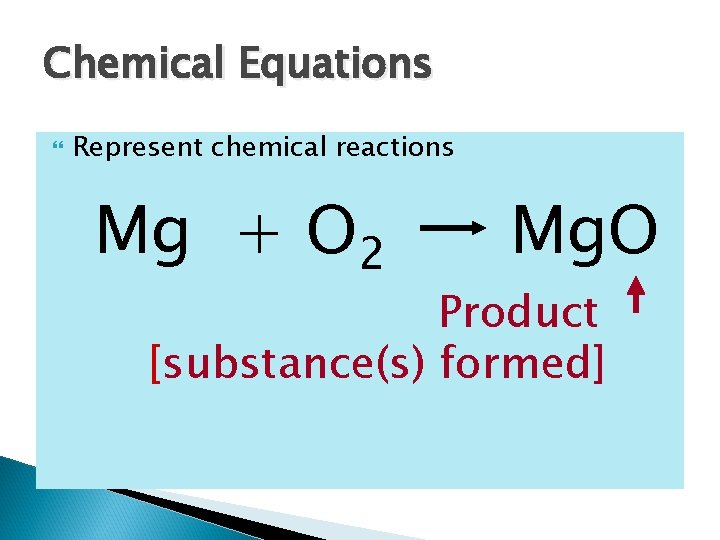Chemical Equations Represent chemical reactions Mg + O 2 Mg. O Product [substance(s) formed]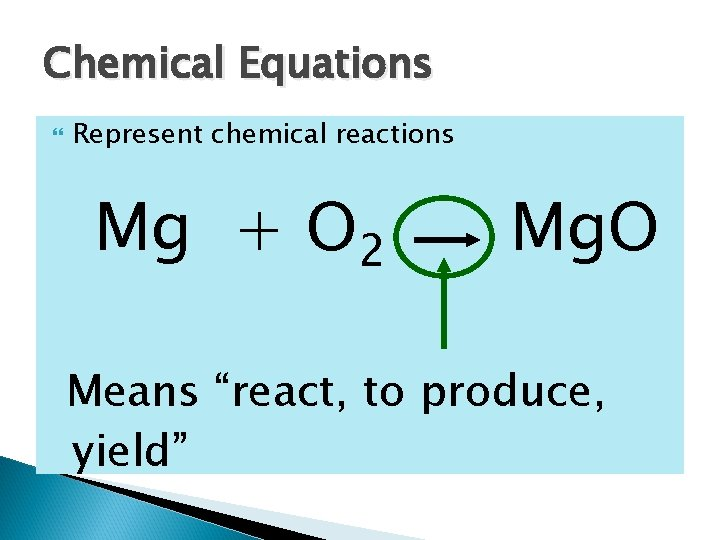Chemical Equations Represent chemical reactions Mg + O 2 Mg. O Means “react, to produce, yield”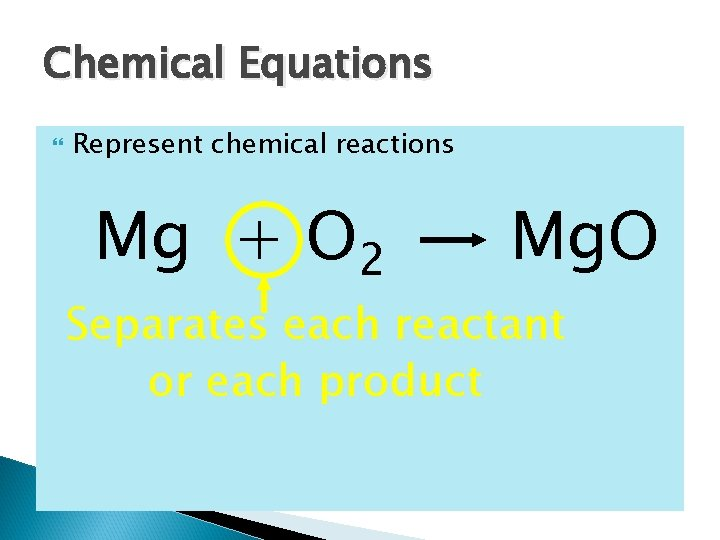Chemical Equations Represent chemical reactions Mg + O 2 Mg. O Separates each reactant or each product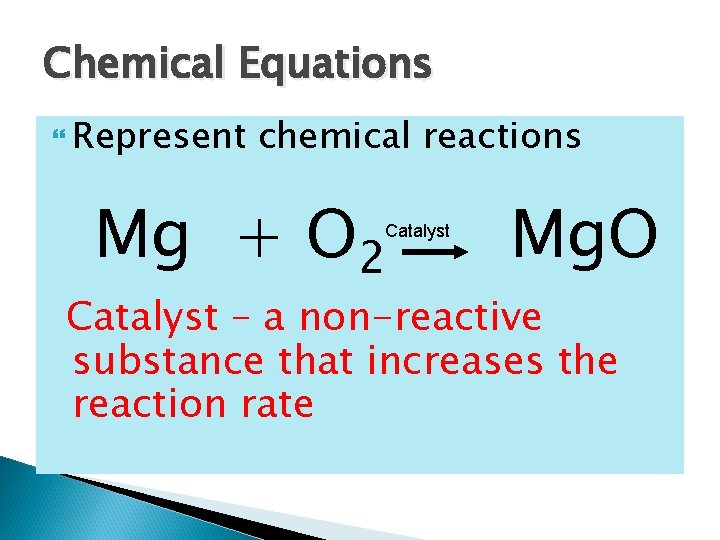Chemical Equations Represent chemical reactions Mg + O 2 Catalyst Mg. O Catalyst – a non-reactive substance that increases the reaction rate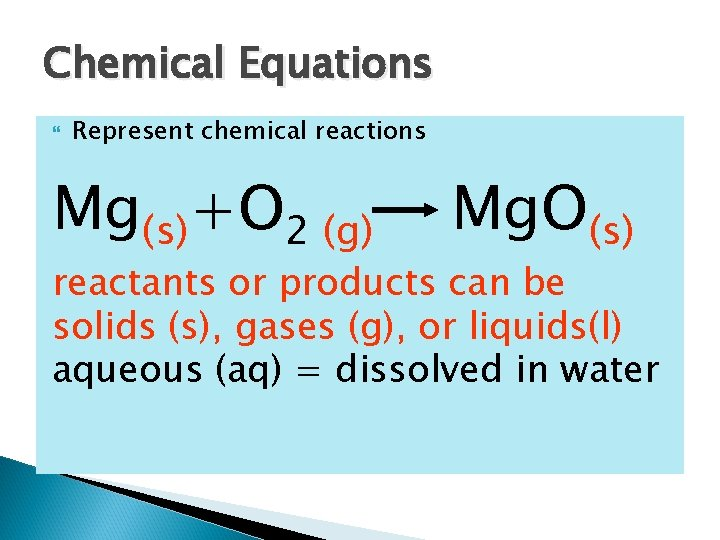Chemical Equations Represent chemical reactions Mg(s)+O 2 (g) Mg. O(s) reactants or products can be solids (s), gases (g), or liquids(l) aqueous (aq) = dissolved in water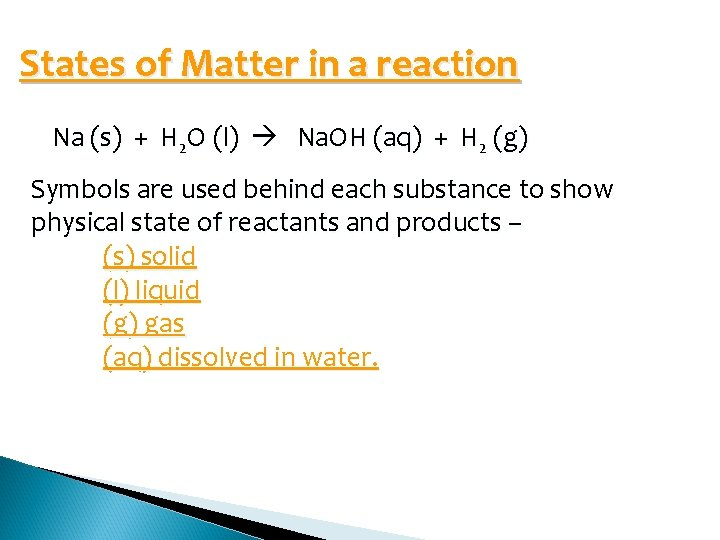States of Matter in a reaction Na (s) + H 2 O (l) Na. OH (aq) + H 2 (g) Symbols are used behind each substance to show physical state of reactants and products – (s) solid (l) liquid (g) gas (aq) dissolved in water.States of Matter Elements – state at room temp (look at periodic table) Solids as products are known as precipitates Aqueous products are produced if there was water present as a reactant (either water itself was a reactant or an aqueous solution was a reactant) Use solubility rules to determine state of matter of ionic compoundsStates of Matter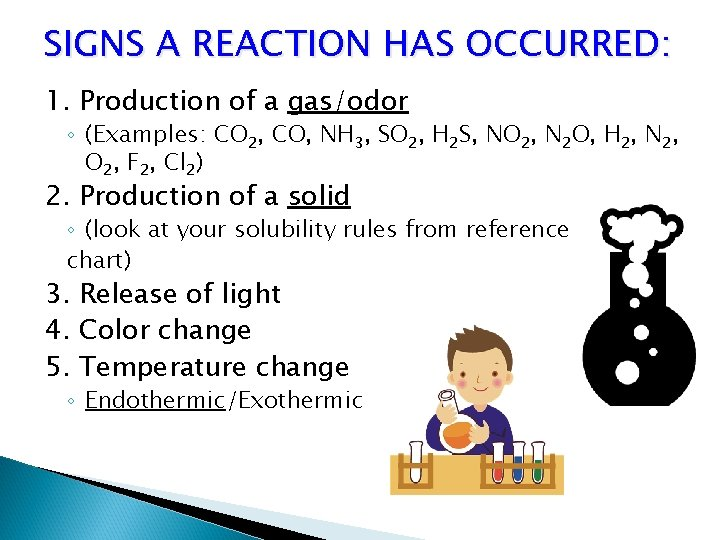SIGNS A REACTION HAS OCCURRED: 1. Production of a gas/odor ◦ (Examples: CO 2, CO, NH 3, SO 2, H 2 S, NO 2, N 2 O, H 2, N 2, O 2, F 2, Cl 2) 2. Production of a solid ◦ (look at your solubility rules from reference chart) 3. Release of light 4. Color change 5. Temperature change ◦ Endothermic/Exothermic5 TYPES OF REACTIONS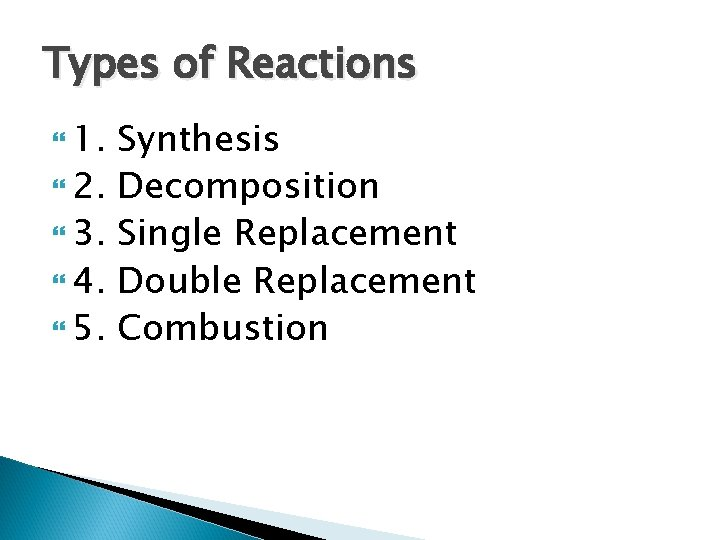Types of Reactions 1. 2. 3. 4. 5. Synthesis Decomposition Single Replacement Double Replacement Combustion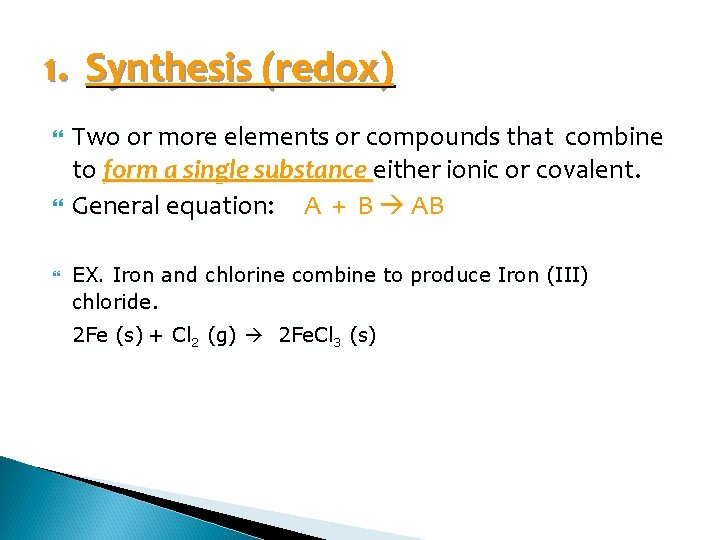1. Synthesis (redox) Two or more elements or compounds that combine to form a single substance either ionic or covalent. General equation: A + B AB EX. Iron and chlorine combine to produce Iron (III) chloride. 2 Fe (s) + Cl 2 (g) 2 Fe. Cl 3 (s)Which of the following equations shows a synthesis reaction? A. Ca. CO 3(s) B. Sb. Cl 3(aq) + H 3 S(aq) C. KBr(aq) + Cl 2(g) D. Al(s) + O 2 (g) Δ Ca. O(s) + CO 2 (g) Sb 3 S 3(s) + HCl(aq) KCl(aq) + Br 2(l) Al 2 O 32. Decomposition A single compound is broken down into two or more substances. Opposite of synthesis. General equation: AB A + B EX. Hydrogen peroxide decomposes into water and oxygen. 2 H 2 O 2 (aq) O 2 (g) + 2 H 2 O (l)Which of the following equations shows a decomposition reaction? A. C 3 H 8 (g) + O 2 (g) CO 2(g) + H 2 O (l) B. KCl. O 3(s) C. Sb. Cl 3(aq) + H 2 S(aq) Sb 2 S 3 D. Mg. O(s) Δ KCl (s) + H 2 O(l) + O 2(g) + HCl(aq) Mg(OH)2(aq)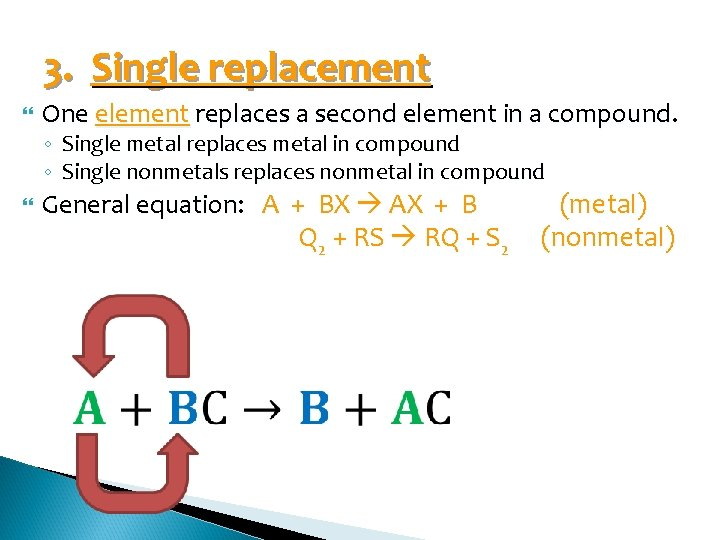3. Single replacement One element replaces a second element in a compound. ◦ ◦ Single metal replaces metal in compound Single nonmetals replaces nonmetal in compound General equation: A + BX AX + B Q 2 + RS RQ + S 2 (metal) (nonmetal)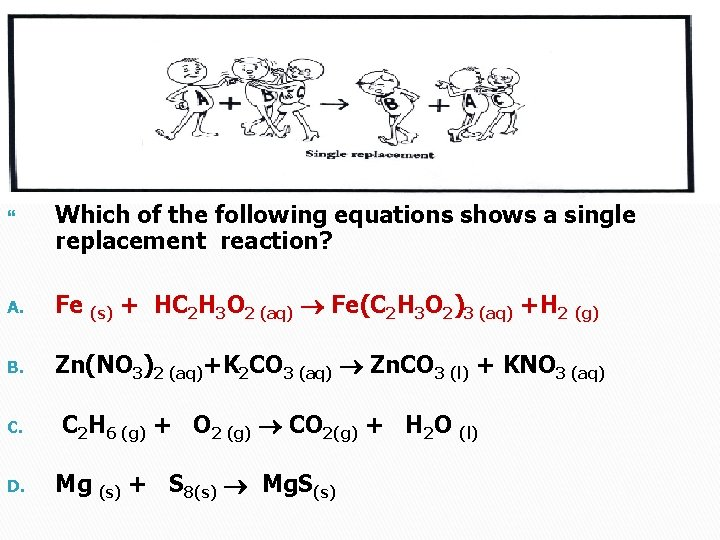Which of the following equations shows a single replacement reaction? A. Fe (s) + HC 2 H 3 O 2 (aq) Fe(C 2 H 3 O 2)3 (aq) +H 2 (g) B. Zn(NO 3)2 (aq)+K 2 CO 3 (aq) Zn. CO 3 (l) + KNO 3 (aq) C. D. C 2 H 6 (g) + O 2 (g) CO 2(g) + H 2 O (l) Mg (s) + S 8(s) Mg. S(s)4. Double replacement Involves an exchange of positive ions between two reacting compounds. Reactants are two ionic compounds AX + BY A Y + B XWhich of the following equations is a double replacement reaction? + KBr B. C 2 H 6 (g) + O 2 (g) CO 2(g) + H 2 O (l) C. Pb. O 2 D. Li(s) + Cl 2 KCl A. + Br 2 HNO 3 Pb(NO 3)4 + H 2 O + O 2 (g) Li 2 O (s)5. Combustion An element or compound reacts with oxygen, often producing energy as heat or light. Cx. Hy. Oz + O 2 CO 2 + H 2 O Some synthesis (combination) reaction fall under this category. General form…you get the oxide of every element you burn (except oxygen). Complete combustion is excess oxygen…gives you CO 2 as the oxide of carbon. Incomplete combustion is limited oxygen…gives you CO as the oxide of carbon.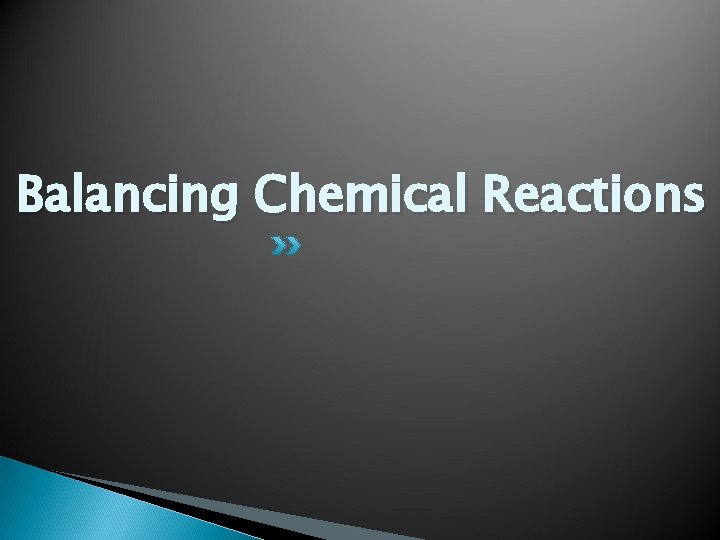Balancing Chemical Reactions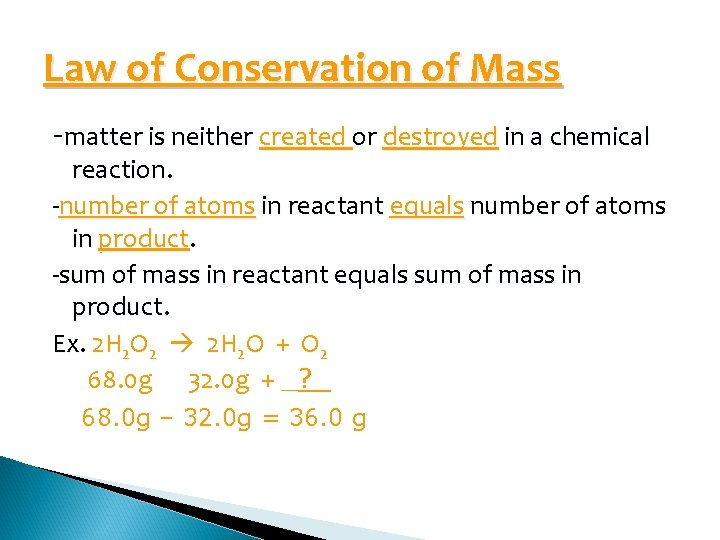Law of Conservation of Mass -matter is neither created or destroyed in a chemical reaction. -number of atoms in reactant equals number of atoms in product. -sum of mass in reactant equals sum of mass in product. Ex. 2 H 2 O 2 2 H 2 O + O 2 68. 0 g 32. 0 g + _? _ 68. 0 g – 32. 0 g = 36. 0 g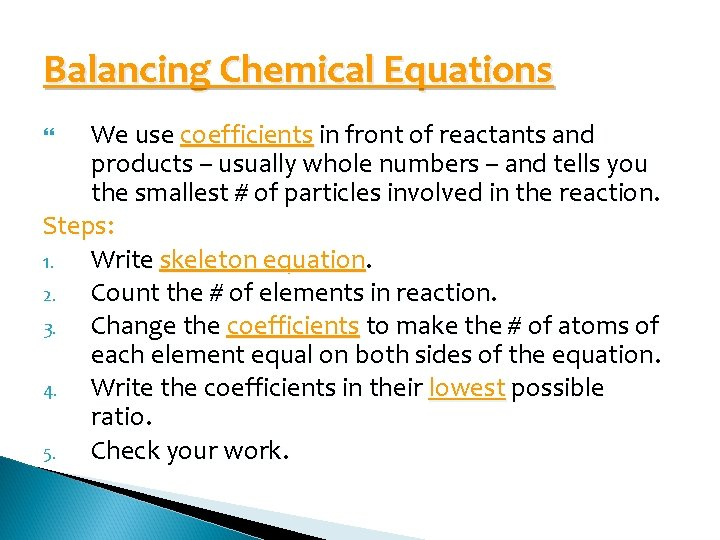Balancing Chemical Equations We use coefficients in front of reactants and products – usually whole numbers – and tells you the smallest # of particles involved in the reaction. Steps: 1. Write skeleton equation. 2. Count the # of elements in reaction. 3. Change the coefficients to make the # of atoms of each element equal on both sides of the equation. 4. Write the coefficients in their lowest possible ratio. 5. Check your work.Helpful Balancing Hints: 1. If an element appears on one side of the reaction more than once, balance it last. Try to make it even on that side. 2. Balancing POLYATOMIC IONS AS A UNIT. 3. Use lowest common factor to balance elements that have an ODD NUMBER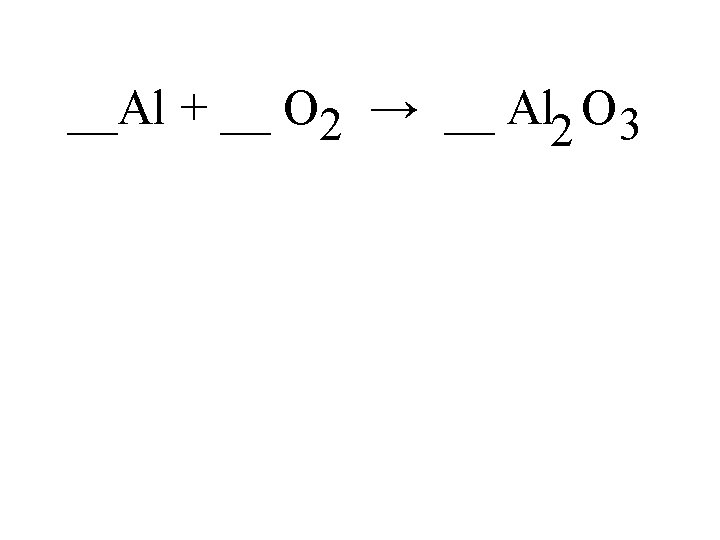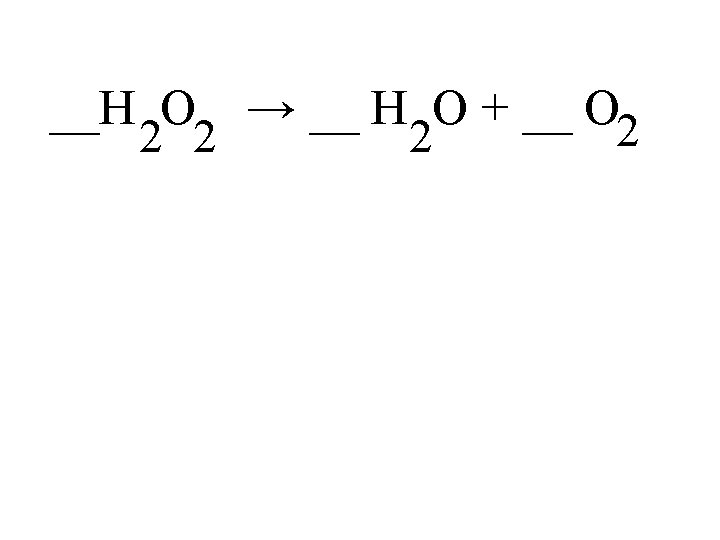__Al + __ H PO 4 → __H + __ Al. PO 3 2 4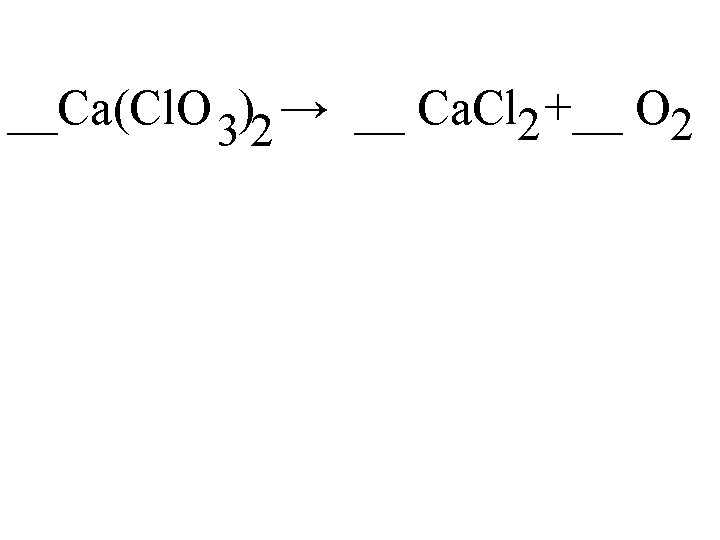__Ca(Cl. O 3)2 → __ Ca. Cl 2 +__ O 2Lets review a few of the Problems: Balance & Types 2 1 3 2 __Fe. Cl 3 + __Be 3(PO 4)2 __Be. Cl 2 + __Fe. PO 4 1 1 __Ag. NO 3 + __Li. OH __Ag. OH + __Li. NO 3 3 1 3 2 __Mg + __Mn 2 O 3 __Mg. O + __MnEQUATION WRITINGWord Equations: Things to Remember Elements: Diatomic or not? Names of common covalent molecules Recognizing if something is ionic or covalent Ionic compounds ◦ Drop and Swap!!! ◦ Transition metals (roman numerals) ◦ Polyatomic ions (endings of names –ide/-ate/-ite)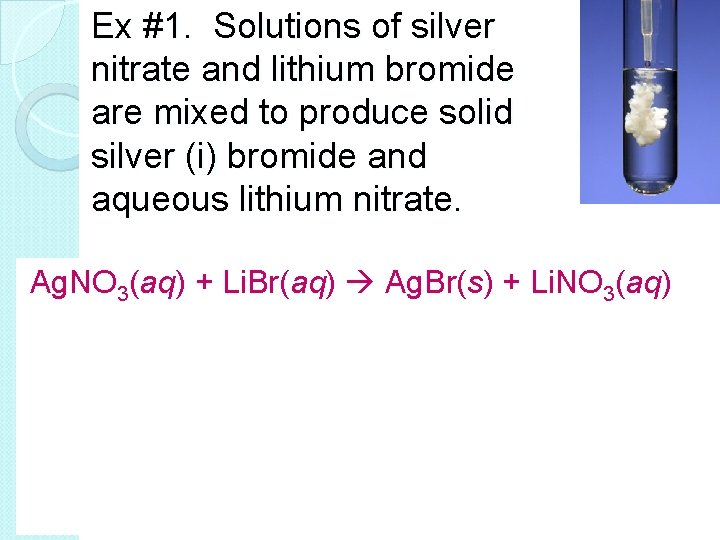Ex #1. Solutions of silver nitrate and lithium bromide are mixed to produce solid silver (i) bromide and aqueous lithium nitrate. Ag. NO 3(aq) + Li. Br(aq) Ag. Br(s) + Li. NO 3(aq)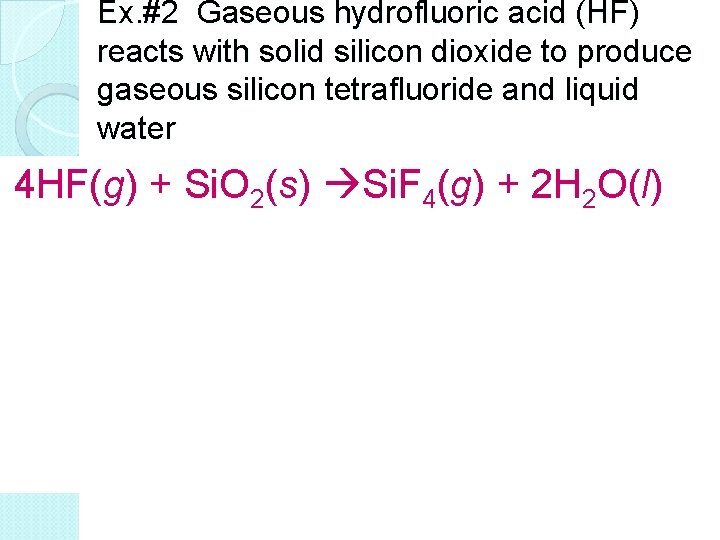Ex. #2 Gaseous hydrofluoric acid (HF) reacts with solid silicon dioxide to produce gaseous silicon tetrafluoride and liquid water 4 HF(g) + Si. O 2(s) Si. F 4(g) + 2 H 2 O(l)Write, Balance and Identify Type on your Board! Silver sulfate + aluminum chloride → aluminum sulfate + silver chloride 3 Ag 2 SO 4 + 2 Al. Cl 3 → Al 2(SO 4)3 + 6 Ag. Cl Double ReplacementWrite, Balance and Identify Type on your Board! Combustion of methane producing carbon dioxide and water CH 4 + 2 O 2 → CO 2 + 2 H 2 O Combustion (limited or complete? )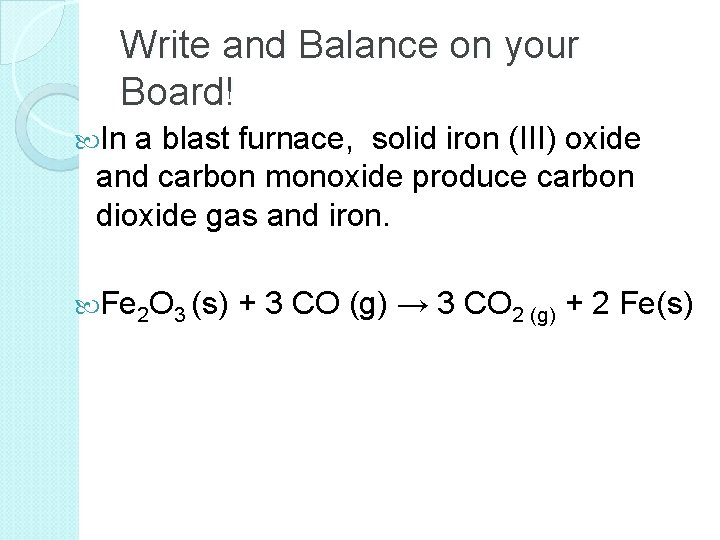Write and Balance on your Board! In a blast furnace, solid iron (III) oxide and carbon monoxide produce carbon dioxide gas and iron. Fe 2 O 3 (s) + 3 CO (g) → 3 CO 2 (g) + 2 Fe(s)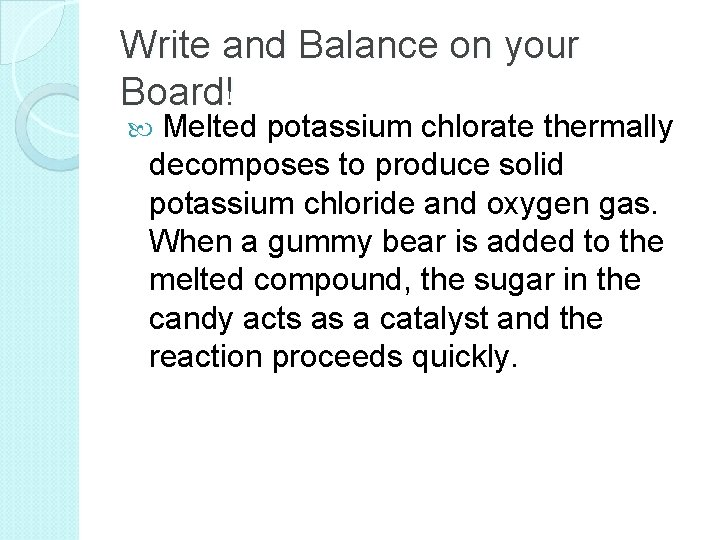Write and Balance on your Board! Melted potassium chlorate thermally decomposes to produce solid potassium chloride and oxygen gas. When a gummy bear is added to the melted compound, the sugar in the candy acts as a catalyst and the reaction proceeds quickly.Packet PracticePacket Practice pg. 9 1. Solid iron reacts with oxygen gas to produce iron (III) oxide. Write the balanced equation then tell how the rust could be prevented from forming. 2. An aqueous solution of hydrogen peroxide will decompose over time to form liquid water and oxygen gas. A solution to disinfect contact lenses utilizes this process but needs to happen overnight so the contacts are ready to be worn in the morning. A catalyst of manganese (IV) dioxide is used to speed up the decomposition.Packet Practice pg. 9 3. In the first step in the production of cement, limestone rock (calcium carbonate) is crushed and heated to 900 o. C to form quicklime (calcium oxide) and carbon dioxide gas. 4. Water is added to the quicklime to get slaked lime (calcium hydroxide) which is used to make plaster. When mixed with sand the result is mortar and if gravel is added, cement forms. Write the equation for the reaction between calcium oxide and water that results in calcium hydroxide.Reaction in a Bag Lab You will perform this lab at your desk in groups of TWO. You DO NOT NEED goggles for this lab. The materials you need for this lab are located on the back lab table. Follow the procedures EXACTLY (I will give them to you after we discuss the lab), making sure you are writing down DETAILED observations as you move through each step of the lab on a piece of paper (1 per student) When the reaction has stopped and you have written down all observations, you can throw the bag away in the trash can. BUT KEEP THE CUP. Clean up your desk, wash out the cup, and put the materials back on the lab station)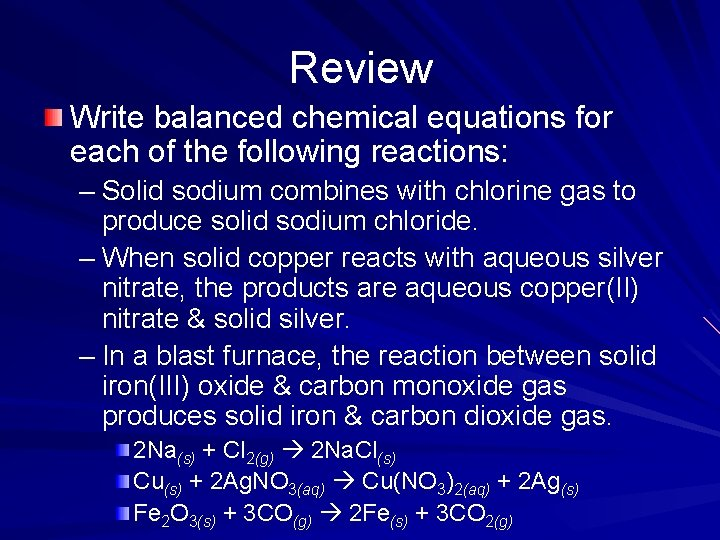Review Write balanced chemical equations for each of the following reactions: – Solid sodium combines with chlorine gas to produce solid sodium chloride. – When solid copper reacts with aqueous silver nitrate, the products are aqueous copper(II) nitrate & solid silver. – In a blast furnace, the reaction between solid iron(III) oxide & carbon monoxide gas produces solid iron & carbon dioxide gas. 2 Na(s) + Cl 2(g) 2 Na. Cl(s) Cu(s) + 2 Ag. NO 3(aq) Cu(NO 3)2(aq) + 2 Ag(s) Fe 2 O 3(s) + 3 CO(g) 2 Fe(s) + 3 CO 2(g)